Next: Confounds Up: tr01cb1 Previous: Estimation

# Model Equivalence

Theorem:

The two-level model specified by equations 1 and 6 is fully equivalent to the single-level model specified by equation 4 in terms of both modelling and estimation when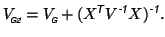(10)

That is, the second-level covariance is set as the sum of the group-covariance from the single-level model and the first-level parameter covariance from the two-level model.

Proof:

We employ the Sherman-Morrison-Woodbury formula (equation 17 from the appendix)

to write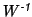as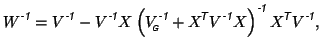(11)

and let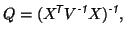which is the covariance estimate for the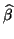s, from equation 5.

Then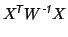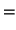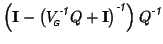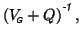by using equation 17 again, and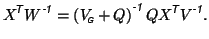Inserting this into equation 8 gives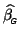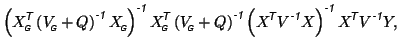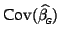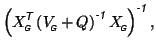which becomes equivalent to equation 9 if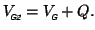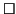For this choice of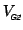, the group-level parameter estimates can be written as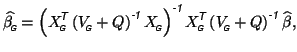that is, they become a function of the first-level parameter estimatesand their associated covariances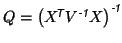only.

Note that by simply applying this theorem multiple times, these results extend to any multi-level GLM. For example, one can calculate parameter estimates for groups of groups using a multi-level approach by only keeping track of parameter estimates and associated covariances at each level. Note also that parts of this proof can simply be obtained by characterising the necessary conditions on sufficient statistics for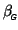.Next: Confounds Up: tr01cb1 Previous: Estimation
Christian Beckmann 2003-07-16